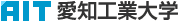日本語
 Please use this identifier to cite or link to this item: http://hdl.handle.net/11133/175

 Title: L^<(m)>_p近似について Other Titles: L^<(m)>_p キンジ ニツイテOn the L^<(m)>_p Approximation Authors: 皆福, 正彦KAIFUKU, Masahiko Issue Date: 30-Dec-1967 Publisher: 愛知工業大学 Abstract: Our paper is devoted to the theoritical and the practical approach for approximating given practical functions or data with the theory of functional vector normed space, particulary L_p^<(m)> norm. As a given function f_1 belongs to the one (functional) space F_1 : f_1∈F_1, and we want to approximate the f_1 with the other function f_2, which is belongs F_2 : f_2∈f_2. Two methods will be possible to approach this problem in the present case ; i.e. the one of which is that, at first, finding the third functional space F_3, such that f_1,f_2∈F_3 and .F_3⊂F_1∩F_2, using the defined norm ∥・∥_ on the space F_3, and function f_3, such as the minimizing the distance d(f_1,f_3), should be search out. the other is the procedure that, if F_1∩F_2=φ (i.e., null space), find out the third space F_3 : F_3⊃F_1∪F_2, and using the norm, difined on the space Fs, the same procedure as the first one would be done. Above all, particullary the importance of Banach, and Hilbert space will be pointed out in the section of the paper. URI: http://hdl.handle.net/11133/175 Appears in Collections: 03号

Files in This Item:

File Description SizeFormatDSpace Software Copyright © 2002-2010  Duraspace - Feedback